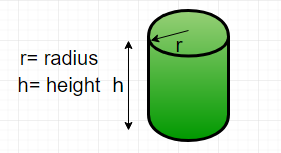# Find the perimeter of a cylinder

• Difficulty Level : Easy
• Last Updated : 22 Jun, 2022

Given diameter and height, find the perimeter of a cylinder.
Perimeter is the length of the outline of a two – dimensional shape. A cylinder is a three – dimensional shape. So, technically we cannot find the perimeter of a cylinder but we can find the perimeter of the cross-section of the cylinder. This can be done by creating the projection on its base, thus, creating the projection on its side, then the shape would be reduced to a rectangle.Formula :
Perimeter of cylinder ( P ) =here d is the diameter of the cylinder
h is the height of the cylinder
Examples :

```Input : diameter = 5, height = 10
Output : Perimeter = 30

Input : diameter = 50, height = 150
Output : Perimeter = 400```

## C++

 `// CPP program to find``// perimeter of cylinder``#include ``using` `namespace` `std;` `// Function to calculate perimeter``int` `perimeter(``int` `diameter, ``int` `height)``{``    ``return` `2 * (diameter + height);``}` `// Driver function``int` `main()``{``    ``int` `diameter = 5;``    ``int` `height = 10;``    ` `    ``cout << ``"Perimeter = "``;``    ``cout<< perimeter(diameter, height);``    ``cout<<``" units\n"``;``    ` `    ``return` `0;``}`

## Java

 `// Java program to find``// perimeter of cylinder``import` `java.io.*;` `class` `GFG {` `    ``// Function to calculate perimeter``    ``static` `int` `perimeter(``int` `diameter, ``int` `height)``    ``{``        ``return` `2` `* (diameter + height);``    ``}``    ` `    ``/* Driver program to test above function */``    ``public` `static` `void` `main(String[] args)``    ``{``        ``int` `diameter = ``5``;``        ``int` `height = ``10``;``        ``System.out.println(``"Perimeter = "` `+``                         ``perimeter(diameter, height)``                                       ``+ ``" units\n"``);``    ``}``}` `// This code is contributed by Gitanjali.`

## Python

 `# Function to calculate``# the perimeter of a cylinder``def` `perimeter( diameter, height ) :``    ``return` `2` `*` `( diameter ``+` `height )` `# Driver function``diameter ``=` `5` `;``height ``=` `10` `;``print` `(``"Perimeter = "``,``            ``perimeter(diameter, height))`

## C#

 `// C# program to find perimeter of cylinder``using` `System;` `class` `GFG {` `    ``// Function to calculate perimeter``    ``static` `int` `perimeter(``int` `diameter, ``int` `height)``    ``{``        ``return` `2 * (diameter + height);``    ``}``    ` `    ``/* Driver program to test above function */``    ``public` `static` `void` `Main(String[] args)``    ``{``        ``int` `diameter = 5;``        ``int` `height = 10;``        ``Console.Write(``"Perimeter = "` `+``                       ``perimeter(diameter, height)``                                    ``+ ``" units\n"``);``    ``}``}` `// This code is contributed by parashar...`

## PHP

 ``

## Javascript

 ``

Output :

`Perimeter = 30 units`

Time Complexity: O(1)
Auxiliary Space: O(1)

My Personal Notes arrow_drop_up